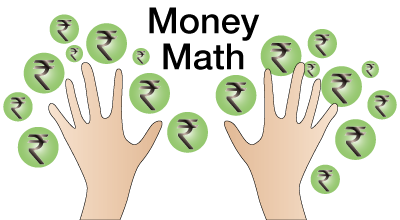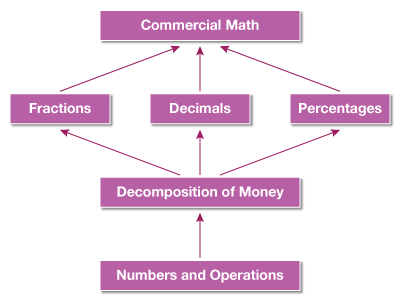In the verge of coronavirus pandemic, we are providing FREE access to our entire Online Curriculum to ensure Learning Doesn't STOP!

# Commercial Math

Go back to  'Maths'

## Introduction to Commercial Math

### Money is what makes the world go round, and mathematics is the centripetal force that governs it. From simple transactions to banking, taxes and discounts, everything that involves money, involves math!## What is Commercial Math?

The branch of mathematics that is used in everyday transactions. These could be in terms of money transactions, business transactions. It lays the base for a lot of related concepts applied concepts like banking, financial planning, investments. Commercial maths help develop business acumen.

## Topics closely related to Commercial Math

The image given below shows what are some of the topics that children should be proficient with to excel or understand commercial maths. For example, a good understanding of decimals, percentages and decomposition of money is important to transact and manage money in real life.## Sub Topics

Given below are the links of all the topics that come under Commerical Math:

## How to Teach Your Child the Commercial Math

The thing to keep in mind is that these are just the beginning. Commercial Math grows in to influence entire fields of study like economics, accountancy, banking. Essentially, once you have a grasp of the basics of commercial math, the entire economic world is your oyster.

1. Allot a game time. Let children engage and learn from games like Monopoly and business.

2. Expose them to the ideas of savings and using their pocket money

3. Ask simple questions about real-life scenarios. For example - how much discount did they get on shopping a certain garment?

## Formulae:

### Simple Interest Formulae:

\begin{align}&P=\text{Principal Amount,} \\ &R=\text{Rate of Interest,} \\ &T=\text{Time Period,} \\ &S\,I=\text{Simple Interest}\end{align}

\begin{align}S\,I=\frac{P\times R\times T}{100}\end{align}

\begin{align}P=\frac{100\times S\,I}{R\times T}\end{align}

\begin{align}R=\frac{100\times S\,I}{P\times T}\end{align}

\begin{align}T=\frac{100\times S\,I}{P\times R}\end{align}

### Compound Interest Formulae:

\begin{align}&P=\text{Principal Amount,} \\ &R=\text{Rate of Interest,} \\ &T=\text{Time Period,} \\ &S\,I=\text{Simple Interest}\end{align}

\begin{align}C\,I=P{{\left( 1+\frac{R}{100} \right)}^{T}}\end{align}

### Profit and Loss Percentage:

\begin{align} Gain \,\%=\left( \frac{\ Gain \times 100\ }{Cost\ Price} \right) \end{align}

\begin{align} Loss \,\% =\left( \frac{\ Loss\times 100}{Cost\ Price} \right) \end{align}

\begin{align} Selling\ Price=\frac{(100+Gain \,\%)}{Cost\ Price}\times (Cost\ Price) \end{align}

\begin{align} Selling\ Price=\frac{(100-Loss\,\%)}{100}\times (Cost\ Price) \end{align}

\begin{align} Cost\ Price=\frac{(100\times Selling\ Price)}{\left( 100+Gain\,\% \right)} \end{align}

\begin{align} Cost\ Price=\frac{(100\times Selling\ Price)}{\left( 100-Loss\,\% \right)} \end{align}